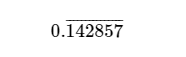# [North Central NA Contest 2018] Rational Ratio | 规律 细节模拟构造 同时被 2 个专栏收录3 篇文章 0 订阅

## Description

Every (positive) rational number can be expressed as a ratio of two (positive) integers. However, in decimal form, rational numbers often have an infifinitely repeating pattern, e.g., 1/7 = 0.142857142857142857… A convenient way of writing this repeating pattern is to put a bar over the fifirst occurrence of the repeating part, so 1/7 would be written:Given a rational number consisting of a series of digits, followed by a decimal point, followed by more digits, and then a number indicating how many of the rightmost digits repeat (i.e., the number of digits under the bar), your task is to find the ratio of two integers, in the most reduced form, that represent the same rational number. For example, for the input “0.142857 6” you should fifind 1/7.

## Input

The input will be a single line with two numbers separated by one space. The fifirst number will consist of 1 to 3 digits (0–9), followed by a decimal point, followed by 1 to 11 digits (0–9), representing the decimal form of the number, possibly with leading zeros. The second number will be a positive integer indicating how many of the rightmost digits of the preceding number repeat. The fifirst number will always be greater than 0. The second number will never be less than 1 nor larger than the number of digits to the right of the decimal point.

## Output

Print the corresponding fraction in its most reduced form, that is, the fraction with the smallest possible integer values in the numerator and denominator.

## Samples

Input Copy

0.142857 6


Output

1/7


Input Copy

1.6 1


Output

5/3


Input Copy

123.456 2


Output

61111/495


[该规律将附到做题总结第26条] 传送门

a b c d e f / 999999 abcdef/999999 ，循环节的长度是多少，就除以一个长度为多少的每位为9的数

string s, t;
int n, flag;
ll zheng, pre, jie;
ll divid;
int siz;
int main() {
cin >> s >> n;
int len = s.size();
for (int i = 0; i < len; i++) {
if (s[i] == '.') {
flag = 1;
continue;
}
if (!flag) {
zheng *= 10;
zheng += s[i] - '0';
}
else {
t.push_back(s[i]);
}
}
int sizFen = t.size();
for (int i = sizFen - n; i < sizFen; i++) {
jie *= 10;
jie += t[i] - '0';
}
int lenPre = sizFen - n;
for (int i = 0; i < sizFen - n; i++) {
pre *= 10;
pre += t[i] - '0';
}
for (int i = 1; i <= lenPre; i++) {
zheng *= 10;
}
zheng += pre;

ll fenzi = 0, fenmu = 0;
fenzi = jie;
for (int i = 1; i <= n; i++) {
fenmu *= 10;
fenmu += 9;
}
fenzi += zheng * fenmu;
//right pos:
for (int i = 1; i <= lenPre; i++) {
fenmu *= 10;
}
ll gd = gcd(fenzi, fenmu);
cout << fenzi / gd << '/' << fenmu / gd << endl;
return 0;
}
/**

**/

10-1110
04-3032105-06454
05-02321
09-083万+
03-122万+
05-064163
11-1615万+
07-282510
11-27205
11-27249
11-27408
11-28107
11-26284
11-2885
11-2894
11-24655
11-231577
11-2539点击重新获取扫码支付余额充值